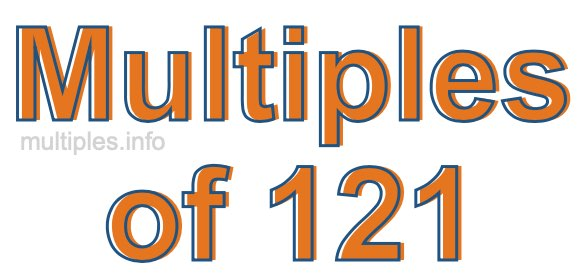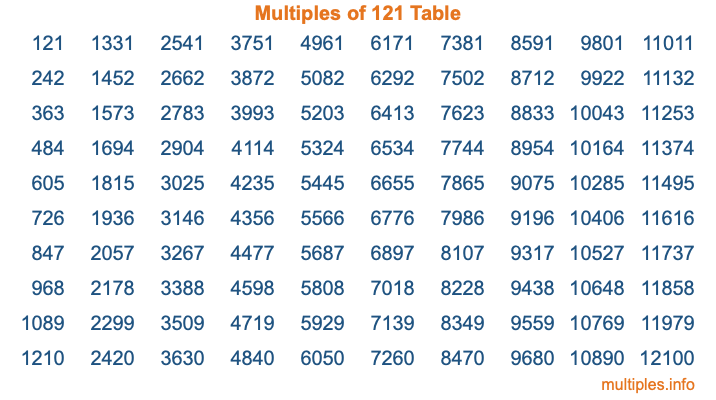Multiples of 121Welcome to the Multiples of 121 page. Here we will first teach you everything you will ever need to know about the multiples of 121, and then give you a study guide summary of everything we taught you to make sure you remember it all. Use this page to look up facts and learn information about the multiples of 121. This page will make you a multiples of one hundred twenty-one expert!

Definition of Multiples of 121
Multiples of 121 are all the numbers that when divided by 121 equal an integer. Each of the multiples of 121 are called a multiple. A multiple of 121 is created by multiplying 121 by an integer.

Therefore, to create a list of multiples of 121, you start with 1 multiplied by 121, then 2 multiplied by 121, then 3 multiplied by 121, and so on for as long as you want. Thus, the list of the first five multiples of 121 is 121, 242, 363, 484, and 605. To see a larger list of multiples of 121, see the printable image of Multiples of 121 further down on this page. We also have a category where you can choose any nth multiple of 121.

Multiples of 121 Checker
The Multiples of 121 Checker below checks to see if any number of your choice is a multiple of 121. In other words, it checks to see if there is any number (integer) that when multiplied by 121 will equal your number. To do that, we divide your number by 121. If the the quotient is an integer, then your number is a multiple of 121.

Is  a multiple of 121?

Least Common Multiple of 121 and ...
A Least Common Multiple (LCM) is the lowest multiple that two or more numbers have in common. This is also called the smallest common multiple or lowest common multiple and is useful to know when you are adding our subtracting fractions. Enter one or more numbers below (121 is already entered) to find the LCM.

Check out our LCM Calculator if you need more details about the Least Common Multiple or if you need the LCM for different numbers for adding and subtraction fractions.

nth Multiple of 121
As we stated above, 121 is the first multiple of 121, 242 is the second multiple of 121, 363 is the third multiple of 121, and so on. Enter a number below to find the nth multiple of 121.

th multiple of 121

Multiples of 121 vs Factors of 121
121 is a multiple of 121 and a factor of 121, but that is where the similarities end. All postive multiples of 121 are 121 or greater than 121. All positive factors of 121 are 121 or less than 121.

Below is the beginning list of multiples of 121 and the factors of 121 so you can compare:

Multiples of 121: 121, 242, 363, 484, 605, etc.

Factors of 121: 1, 11, 121

As you can see, the multiples of 121 are all the numbers that you can divide by 121 to get a whole number. The factors of 121, on the other hand, are all the whole numbers that you can multiply by another whole number to get 121.

It's also interesting to note that if a number (x) is a factor of 121, then 121 will also be a multiple of that number (x).

Multiples of 121 vs Divisors of 121
The divisors of 121 are all the integers that 121 can be divided by evenly. Below is a list of the divisors of 121.

Divisors of 121: 1, 11, 121

The interesting thing to note here is that if you take any multiple of 121 and divide it by a divisor of 121, you will see that the quotient is an integer.

Multiples of 121 Table
Below is an image of the first 100 multiples of 121 in a table. The table is in chronological order, column by column. The first column has the first ten multiples of 121, the second column has the next ten multiples of 121, and so on.The Multiples of 121 Table is also referred to as the 121 Times Table or Times Table of 121. You are welcome to print out our table for your studies.

Negative Multiples of 121
Although not often discussed or needed in math, it is worth mentioning that you can make a list of negative multiples of 121 by multiplying 121 by -1, then by -2, then by -3, and so on, to get the following list of negative multiples of 121:

-121, -242, -363, -484, -605, etc.

Multiples of 121 Summary
Below is a summary of important Multiples of 121 facts that we have discussed on this page. To retain the knowledge on this page, we recommend that you read through the summary and explain to yourself or a study partner why they hold true.

There are an infinite number of multiples of 121.

A multiple of 121 divided by 121 will equal a whole number.

121 divided by a factor of 121 equals a divisor of 121.

The nth multiple of 121 is n times 121.

The largest factor of 121 is equal to the first positive multiple of 121.

121 is a multiple of every factor of 121.

121 is a multiple of 121.

A multiple of 121 divided by a divisor of 121 equals an integer.

121 divided by a divisor of 121 equals a factor of 121.

Any integer times 121 will equal a multiple of 121.

Multiples of a Number
Here you can get the multiples of another number, all with the same attention to detail as we did for multiples of 121 on this page.

Multiples of
Multiples of 122
Did you find our page about multiples of one hundred twenty-one educational? Do you want more knowledge? Check out the multiples of the next number on our list!